## Paging in OS-

Before you go through this article, make sure that you have gone through the previous articles on Paging in OS.

We have discussed-

• Paging is a non-contiguous memory allocation technique.
• Page Table is a table that maps a page number to the frame number containing that page.
• Multilevel Paging is a paging scheme where there exists a hierarchy of page tables.
• Translation Lookaside Buffer tries to reduce the effective access time.

Also Read- Important Formulas of Paging

In this article, we will discuss practice problems based on multilevel paging using TLB.

## Effective Access Time-

In a multilevel paging scheme using TLB, the effective access time is given by-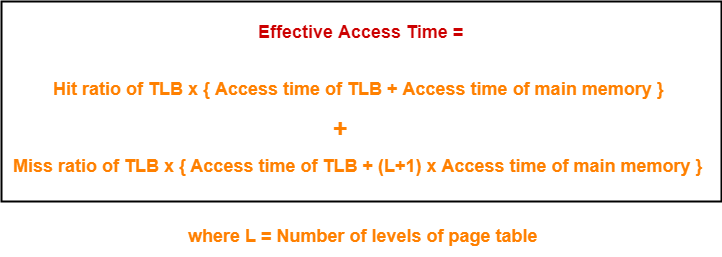This formula is valid only when there are no Page Faults.

## Problem-01:

Consider a single level paging scheme with a TLB. Assume no page fault occurs. It takes 20 ns to search the TLB and 100 ns to access the physical memory. If TLB hit ratio is 80%, the effective memory access time is _______ msec.

## Solution-

Given-

• Number of levels of page table = 1
• TLB access time = 20 ns
• Main memory access time = 100 ns
• TLB Hit ratio = 80% = 0.8

### Calculating TLB Miss Ratio-

TLB Miss ratio

= 1 – TLB Hit ratio

= 1 – 0.8

= 0.2

### Calculating Effective Access Time-

Substituting values in the above formula, we get-

Effective Access Time

= 0.8 x { 20 ns + 100 ns } + 0.2 x { 20 ns + (1+1) x 100 ns }

= 0.8 x 120 ns + 0.2 + 220 ns

= 96 ns + 44 ns

= 140 ns

Thus, effective memory access time = 140 ns.

## Problem-02:

Consider a two level paging scheme with a TLB. Assume no page fault occurs. It takes 20 ns to search the TLB and 100 ns to access the physical memory. If TLB hit ratio is 80%, the effective memory access time is _______ msec.

## Solution-

Given-

• Number of levels of page table = 2
• TLB access time = 20 ns
• Main memory access time = 100 ns
• TLB Hit ratio = 80% = 0.8

### Calculating TLB Miss Ratio-

TLB Miss ratio

= 1 – TLB Hit ratio

= 1 – 0.8

= 0.2

### Calculating Effective Access Time-

Substituting values in the above formula, we get-

Effective Access Time

= 0.8 x { 20 ns + 100 ns } + 0.2 x { 20 ns + (2+1) x 100 ns }

= 0.8 x 120 ns + 0.2 + 320 ns

= 96 ns + 64 ns

= 160 ns

Thus, effective memory access time = 160 ns.

## Problem-03:

Consider a three level paging scheme with a TLB. Assume no page fault occurs. It takes 20 ns to search the TLB and 100 ns to access the physical memory. If TLB hit ratio is 80%, the effective memory access time is _______ msec.

## Solution-

Given-

• Number of levels of page table = 3
• TLB access time = 20 ns
• Main memory access time = 100 ns
• TLB Hit ratio = 80% = 0.8

### Calculating TLB Miss Ratio-

TLB Miss ratio

= 1 – TLB Hit ratio

= 1 – 0.8

= 0.2

### Calculating Effective Access Time-

Substituting values in the above formula, we get-

Effective Access Time

= 0.8 x { 20 ns + 100 ns } + 0.2 x { 20 ns + (3+1) x 100 ns }

= 0.8 x 120 ns + 0.2 + 420 ns

= 96 ns + 84 ns

= 180 ns

Thus, effective memory access time = 180 ns.

## Problem-04:

Consider a single level paging scheme with a TLB. Assume no page fault occurs. It takes 20 ns to search the TLB and 100 ns to access the physical memory. If effective memory access time is 130 ns, TLB hit ratio is ______.

## Solution-

Given-

• Number of levels of page table = 1
• TLB access time = 20 ns
• Main memory access time = 100 ns
• Effective memory access time = 130 ns

Let TLB Hit ratio = H

### Calculating TLB Miss Ratio-

TLB Miss ratio

= 1 – TLB Hit ratio

= 1 – H

### Calculating TLB Hit Ratio-

Substituting values in the above formula, we get-

130 ns = H x { 20 ns + 100 ns } + (1-H) x { 20 ns + (1+1) x 100 ns }

130 ns = H x { 120 ns } + (1-H) x { 220 ns }

130 ns = 120H ns + 220 ns – 220H ns

220H ns – 120H ns = 220 ns – 130 ns

100H ns = 90 ns

H = 90 / 100

∴ H = 0.9

Thus, TLB hit ratio = 0.9 or 90%.

## Problem-05:

Consider a single level paging scheme with a TLB. Assume no page fault occurs. It takes 100 ns to access the physical memory. If TLB hit ratio is 60% and effective memory access time is 130 ns, TLB access time is ______.

## Solution-

Given-

• Number of levels of page table = 1
• Main memory access time = 100 ns
• TLB Hit ratio = 60% = 0.6
• Effective memory access time = 160 ns

Let TLB access time = T ns

### Calculating TLB Miss Ratio-

TLB Miss ratio

= 1 – TLB Hit ratio

= 1 – 0.6

= 0.4

### Calculating TLB Access Time-

Substituting values in the above formula, we get-

160 ns = 0.6 x { T ns + 100 ns } + 0.4 x { T ns + (1+1) x 100 ns }

160 ns = 0.6 x { T ns + 100 ns } + 0.4 x { T ns + 200 ns }

160 ns = 0.6T ns + 60 ns + 0.4T ns + 80 ns

0.6T ns + 0.4T ns = 160 ns – 60 ns – 80 ns

T ns = 20 ns

∴ T = 20

Thus, TLB access time = 20 ns.

## Problem-06:

Consider a single level paging scheme with a TLB. Assume no page fault occurs. It takes 20 ns to search the TLB. If TLB hit ratio is 50% and effective memory access time is 170 ns, main memory access time is ______.

## Solution-

Given-

• Number of levels of page table = 1
• TLB access time = 20 ns
• TLB Hit ratio = 50% = 0.5
• Effective memory access time = 170 ns

Let main memory access time = T ns

### Calculating TLB Miss Ratio-

TLB Miss ratio

= 1 – TLB Hit ratio

= 1 – 0.5

= 0.5

### Calculating Main Memory Access Time-

Substituting values in the above formula, we get-

170 ns = 0.5 x { 20 ns + T ns } + 0.5 x { 20 ns + (1+1) x T ns }

170 ns = 0.5 x { 20 ns + T ns } + 0.5 x { 20 ns + 2T ns }

170 ns = 10 ns + 0.5T ns + 10 ns + T ns

0.5T ns + T ns = 170 ns – 10 ns – 10 ns

1.5T ns = 150 ns

T = 150 / 1.5

∴ T = 100

Thus, Main Memory access time = 100 ns.

Next Article- Page Faults in OS

Get more notes and other study material of Operating System.

Watch video lectures by visiting our YouTube channel LearnVidFun.

## Paging in OS-

Before you go through this article, make sure that you have gone through the previous articles on Paging in OS.

We have discussed-

• Paging is a non-contiguous memory allocation technique.
• Page Table is a table that maps a page number to the frame number containing that page.
• Multilevel Paging is a paging scheme where there exists a hierarchy of page tables.

Also Read- Important Formulas of Paging

In this article, we will discuss practice problems based on multilevel paging.

## Problem-01:

Consider a single level paging scheme. The page size is 4 KB and page table entry size is 4 bytes. The size of page table is 4 KB. Give the division of virtual address space.

## Solution-

Given-

• Page size = 4 KB
• Page table entry size = 4 bytes
• Page table size = 4 KB

Let the number of bits in virtual address = n bits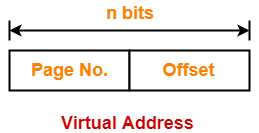### Number of Bits in Page Offset-

We have,

Page size

= 4 KB

= 212 B

Thus, Number of bits in page offset = 12 bits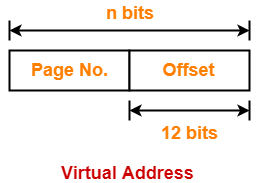### Process Size-

Number of bits in virtual address = n bits

Thus,

Process size = 2n bytes

### Number of Pages of Process-

Number of pages the process is divided

= Process size / Page size

= 2n B / 4 KB

= 2n B / 212 B

= 2n-12 pages

### Page Table Size-

Page table keeps track of the frames storing the pages of process.

Page table size

= Number of entries in page table x Page table entry size

= Number of pages the process is divided x Page table entry size

= 2n-12 x 4 bytes

= 2n-12+2 bytes

= 2n-10 bytes

But we are given page table size = 4 KB

Thus,

2n-10 bytes = 4 KB

2n-10 = 212

n – 10 = 12

∴ n = 22

Thus, number of bits in virtual address = 22 bits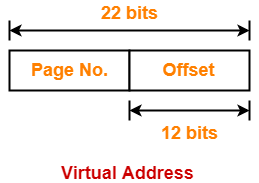### Method-01:

Number of bits required to search a particular entry in page table

= Number of bits in virtual address – Number of bits in page offset

= 22 bits – 12 bits

= 10 bits

### Method-02:

Number of entries in page table

= Number of pages the process is divided

= 2n-12

= 222-12

= 210

Thus, Number of bits required to search a particular entry in page table = 10 bits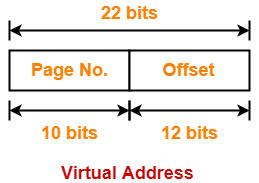## Problem-02:

Consider a two level paging scheme. The page size is 4 KB and page table entry size is 4 bytes. The size of outer page table is 4 KB. Give the division of virtual address space.

## Solution-

Given-

• Page size = 4 KB
• Page table entry size = 4 bytes
• Page table size = 4 KB

Let the number of bits in virtual address = n bits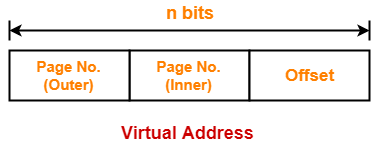### Number of Bits in Page Offset-

We have,

Page size

= 4 KB

= 212 B

Thus, Number of bits in page offset = 12 bits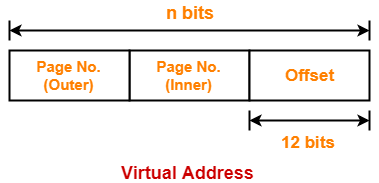### Process Size-

Number of bits in virtual address = n bits

Thus,

Process size = 2n bytes

### Number of Pages of Process-

Number of pages the process is divided

= Process size / Page size

= 2n B / 4 KB

= 2n B / 212 B

= 2n-12 pages

### Inner Page Table Size-

Inner page table keeps track of the frames storing the pages of process.

Inner page table size

= Number of entries in inner page table x Page table entry size

= Number of pages the process is divided x Page table entry size

= 2n-12 x 4 bytes

= 2n-12+2 bytes

= 2n-10 bytes

### Number of Pages of Inner Page Table-

Number of pages the inner page table is divided

= Inner page table size / Page size

= 2n-10 B / 4 KB

= 2n-10 B / 212 B

= 2n-10-12

= 2n-22 pages

Now, these 2n-22 pages of inner page table are stored in different frames of the main memory.

### Number of Page Table Entries in One Page of Inner Page Table-

Number of page table entries in one page of inner page table

= Page size / Page table entry size

= 4 KB / 4 bytes

= 1 K

= 210 entries

### Number of Bits Required to Search an Entry in One Page of Inner Page Table-

One page of inner page table contains 210 entries.

Thus,

Number of bits required to search a particular entry in one page of inner page table = 10 bits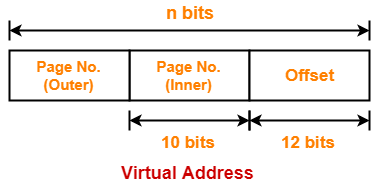### Outer Page Table Size-

Outer page table is required to keep track of the frames storing the pages of inner page table.

Outer page table size

= Number of entries in outer page table x Page table entry size

= Number of pages the inner page table is divided x Page table entry size

= 2n-22 x 4 bytes

= 2n-22+2 bytes

= 2n-20 bytes

But we are given outer page table size = 4 KB

Thus,

2n-20 bytes = 4 KB

2n-20 = 212

n – 20 = 12

∴ n = 32

Thus, number of bits in virtual address = 32 bits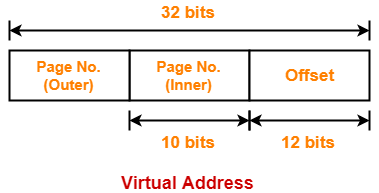### Method-01:

Number of bits required to search a particular entry in outer page table

= Number of bits in virtual address – (Number of bits required to search an entry in inner page table + Number of bits in page offset)

= 32 bits – (10 bits + 12 bits)

= 32 bits – 22 bits

= 10 bits

### Method-02:

Number of entries in outer page table

= Number of pages the inner page table is divided

= 2n-22

= 232-22

= 210

Thus, Number of bits required to search a particular entry in outer page table = 10 bits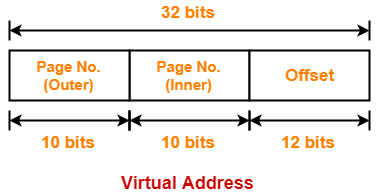## Problem-03:

Consider a three level paging scheme. The page size is 4 KB and page table entry size is 4 bytes. The size of outermost page table is 4 KB. Give the division of virtual address space.

## Solution-

Given-

• Page size = 4 KB
• Page table entry size = 4 bytes
• Page table size = 4 KB

Let the number of bits in virtual address = n bits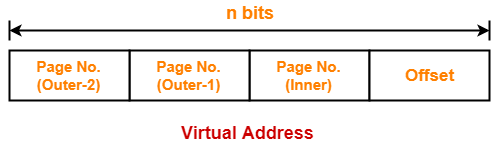### Number of Bits in Page Offset-

We have,

Page size

= 4 KB

= 212 B

Thus, Number of bits in page offset = 12 bits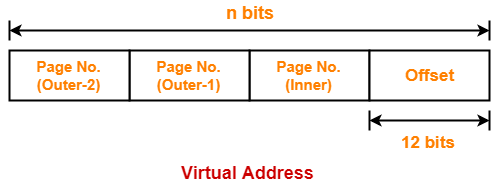### Process Size-

Number of bits in virtual address = n bits

Thus,

Process size = 2n bytes

### Number of Pages of Process-

Number of pages the process is divided

= Process size / Page size

= 2n B / 4 KB

= 2n B / 212 B

= 2n-12 pages

### Inner Page Table Size-

Inner page table keeps track of the frames storing the pages of process.

Inner page table size

= Number of entries in inner page table x Page table entry size

= Number of pages the process is divided x Page table entry size

= 2n-12 x 4 bytes

= 2n-12+2 bytes

= 2n-10 bytes

### Number of Pages of Inner Page Table-

Number of pages the inner page table is divided

= Inner page table size / Page size

= 2n-10 B / 4 KB

= 2n-10 B / 212 B

= 2n-10-12

= 2n-22 pages

Now, these 2n-22 pages of inner page table are stored in different frames of the main memory.

### Number of Page Table Entries in One Page of Inner Page Table-

Number of page table entries in one page of inner page table

= Page size / Page table entry size

= 4 KB / 4 bytes

= 1 K

= 210 entries

### Number of Bits Required to Search an Entry in One Page of Inner Page Table-

One page of inner page table contains 210 entries.

Thus,

Number of bits required to search a particular entry in one page of inner page table = 10 bits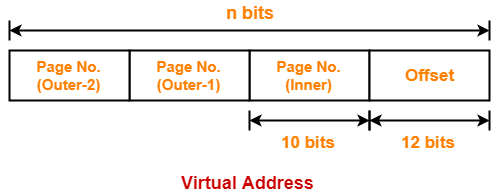### Outer Page Table-1 Size-

Outer page table-1 is required to keep track of the frames storing the pages of inner page table.

Outer page table-1 size

= Number of entries in outer page table-1 x Page table entry size

= Number of pages the inner page table is divided x Page table entry size

= 2n-22 x 4 bytes

= 2n-22+2 bytes

= 2n-20 bytes

### Number of Pages of Outer Page Table-1

Number of pages the outer page table-1 is divided

= Outer page table-1 size / Page size

= 2n-20 B / 4 KB

= 2n-20 B / 212 B

= 2n-20-12

= 2n-32 pages

Now, these 2n-32 pages of outer page table-1 are stored in different frames of the main memory.

### Number of Page Table Entries in One Page of Outer Page Table-1

Number of page table entries in one page of outer page table-1

= Page size / Page table entry size

= 4 KB / 4 bytes

= 1 K

= 210 entries

### Number of Bits Required to Search an Entry in One Page of Outer Page Table-1

One page of outer page table-1 contains 210 entries.

Thus,

Number of bits required to search a particular entry in one page of outer page table-1 = 10 bits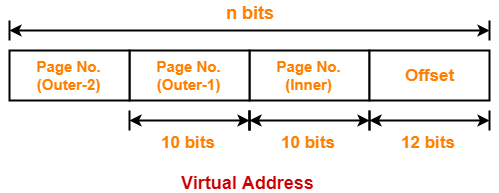### Outer Page Table-2 Size-

Outer page table-2 is required to keep track of the frames storing the pages of outer page table-1.

Outer page table-2 size

= Number of entries in outer page table-2 x Page table entry size

= Number of pages the outer page table-1 is divided x Page table entry size

= 2n-32 x 4 bytes

= 2n-32+2 bytes

= 2n-30 bytes

But we are given outermost page table size = 4 KB

Thus,

2n-30 bytes = 4 KB

2n-30 = 212

n – 30 = 12

∴ n = 42

Thus, number of bits in virtual address = 42 bits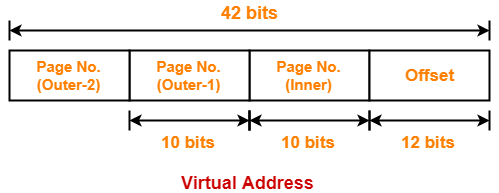### Method-01:

Number of bits required to search a particular entry in outer page table-2

= Number of bits in virtual address – (Number of bits required to search an entry in outer page table-1 + Number of bits required to search an entry in inner page table + Number of bits in page offset)

= 42 bits – (10 bits + 10 bits + 12 bits)

= 42 bits – 32 bits

= 10 bits

### Method-02:

Number of entries in outer page table-2

= Number of pages the outer page table-1 is divided

= 2n-32

= 242-32

= 210

Thus, Number of bits required to search a particular entry in outer page table-2 = 10 bits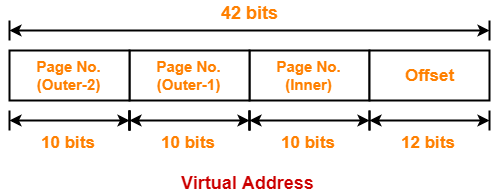## Problem-04:

Complete the following table-

 Page Size Page Table Entry Size Outermost Page Table Size Levels of Paging Virtual Address Space Division 4 KB 4 bytes 256 bytes 1 ? 4 KB 4 bytes 256 bytes 2 ? 4 KB 4 bytes 256 bytes 3 ?

## Solution-

• We have to solve these problems in exactly the same manner as we have solved above.
• So, try yourself.

The answers to these problems are-

1. 6 bits, 12 bits
2. 6 bits, 10 bits, 12 bits
3. 6 bits, 10 bits, 10 bits, 12 bits

Get more notes and other study material of Operating System.

Watch video lectures by visiting our YouTube channel LearnVidFun.

## Paging in OS-

Before you go through this article, make sure that you have gone through the previous articles on Paging in OS.

We have discussed-

• Paging is a non-contiguous memory allocation technique.
• Page Table is a table that maps a page number to the frame number containing that page.

Also Read- Important Formulas of Paging

In this article, we will discuss practice problems based on concepts of paging.

## Problem-01:

Consider a single level paging scheme. The virtual address space is 4 MB and page size is 4 KB. What is the maximum page table entry size possible such that the entire page table fits well in one page?

## Solution-

For page table, to fit well in one page, we must have-

Page table  size <= Page size

### Number of Pages of Process-

Number of pages the process is divided

= Process size / Page size

= 4 MB / 4 KB

= 210 pages

### Page Table Size-

Let page table entry size = B bytes

Now,

Page table size

= Number of entries in the page table x Page table entry size

= Number of pages the process is divided x Page table entry size

= 210 x B bytes

Now,

According to the above condition, we must have-

210 x B bytes <= 4 KB

210 x B <= 212

B <= 4

Thus, maximum page table entry size possible = 4 bytes.

## Problem-02:

Consider a single level paging scheme. The virtual address space is 4 GB and page size is 128 KB. What is the maximum page table entry size possible such that the entire page table fits well in one page?

## Solution-

For page table, to fit well in one page, we must have-

Page table  size <= Page size

### Number of Pages of Process-

Number of pages the process is divided

= Process size / Page size

= 4 GB / 128 KB

= 232 B / 217 B

= 215 pages

### Page Table Size-

Let page table entry size = B bytes

Now,

Page table size

= Number of entries in the page table x Page table entry size

= Number of pages the process is divided x Page table entry size

= 215 x B bytes

Now,

According to the above condition, we must have-

215 x B bytes <= 128 KB

215 x B <= 217

B <= 4

Thus, maximum page table entry size possible = 4 bytes.

## Problem-03:

Consider a single level paging scheme. The virtual address space is 128 TB and page size is 32 MB. What is the maximum page table entry size possible such that the entire page table fits well in one page?

## Solution-

For page table, to fit well in one page, we must have-

Page table  size <= Page size

### Number of Pages of Process-

Number of pages the process is divided

= Process size / Page size

= 128 TB / 32 MB

= 247 B / 225 B

= 222 pages

### Page Table Size-

Let page table entry size = B bytes

Now,

Page table size

= Number of entries in the page table x Page table entry size

= Number of pages the process is divided x Page table entry size

= 222 x B bytes

Now,

According to the above condition, we must have-

222 x B bytes <= 32 MB

222 x B <= 225

B <= 8

Thus, maximum page table entry size possible = 8 bytes.

## Problem-04:

Consider a single level paging scheme. The virtual address space is 256 MB and page table entry size is 4 bytes. What is the minimum page size possible such that the entire page table fits well in one page?

## Solution-

For page table, to fit well in one page, we must have-

Page table  size <= Page size

Let page size = B bytes.

### Number of Pages of Process-

Number of pages the process is divided

= Process size / Page size

= 256 MB / B bytes

= 228 / B

### Page Table Size-

Page table size

= Number of entries in the page table x Page table entry size

= Number of pages the process is divided x Page table entry size

= (228 / B) x 4 bytes

= (230 / B) bytes

Now,

According to the above condition, we must have-

(230 / B) bytes <= B bytes

B2 >= 230

B >= 215

Thus, minimum page size possible = 215 bytes or 32 KB.

## Problem-05:

Consider a single level paging scheme. The virtual address space is 512 KB and page table entry size is 2 bytes. What is the minimum page size possible such that the entire page table fits well in one page?

## Solution-

For page table, to fit well in one page, we must have-

Page table  size <= Page size

Let page size = B bytes.

### Number of Pages of Process-

Number of pages the process is divided

= Process size / Page size

= 512 KB / B bytes

= 219 / B

### Page Table Size-

Page table size

= Number of entries in the page table x Page table entry size

= Number of pages the process is divided x Page table entry size

= (219 / B) x 2 bytes

= (220 / B) bytes

Now,

According to the above condition, we must have-

(220 / B) bytes <= B bytes

B2 >= 220

B >= 210

Thus, minimum page size possible = 210 bytes or 1 KB.

## Problem-06:

Consider a single level paging scheme. The virtual address space is 16 GB and page table entry size is 4 bytes. What is the minimum page size possible such that the entire page table fits well in one page?

## Solution-

For page table, to fit well in one page, we must have-

Page table  size <= Page size

Let page size = B bytes.

### Number of Pages of Process-

Number of pages the process is divided

= Process size / Page size

= 16 GB / B bytes

= 234 / B

### Page Table Size-

Page table size

= Number of entries in the page table x Page table entry size

= Number of pages the process is divided x Page table entry size

= (234 / B) x 4 bytes

= (236 / B) bytes

Now,

According to the above condition, we must have-

(236 / B) bytes <= B bytes

B2 >= 236

B >= 218

Thus, minimum page size possible = 218 bytes or 256 KB.

Next Article- Translation Lookaside Buffer | TLB

Get more notes and other study material of Operating System.

Watch video lectures by visiting our YouTube channel LearnVidFun.

## Paging in OS-

Before you go through this article, make sure that you have gone through the previous article on Paging in OS.

We have discussed-

• Paging in OS is a non-contiguous memory allocation technique.
• Page Table is a data structure that maps page number to the frame number.

One major disadvantage of paging is-

• It increases the effective access time due to increased number of memory accesses.
• One memory access is required to get the frame number from the page table.
• Another memory access is required to get the word from the page.

## Translation Lookaside Buffer-

• Translation Lookaside Buffer (TLB) is a solution that tries to reduce the effective access time.
• Being a hardware, the access time of TLB is very less as compared to the main memory.

## Structure-

Translation Lookaside Buffer (TLB) consists of two columns-

1. Page Number
2. Frame Number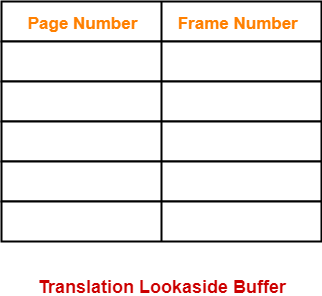In a paging scheme using TLB,

The logical address generated by the CPU is translated into the physical address using following steps-

## Step-01:

CPU generates a logical address consisting of two parts-

1. Page Number
2. Page Offset

## Step-02:

• TLB is checked to see if it contains an entry for the referenced page number.
• The referenced page number is compared with the TLB entries all at once.

Now, two cases are possible-

### Case-01: If there is a TLB hit-

• If TLB contains an entry for the referenced page number, a TLB hit occurs.
• In this case, TLB entry is used to get the corresponding frame number for the referenced page number.

### Case-02: If there is a TLB miss-

• If TLB does not contain an entry for the referenced page number, a TLB miss occurs.
• In this case, page table is used to get the corresponding frame number for the referenced page number.
• Then, TLB is updated with the page number and frame number for future references.

## Step-03:

• After the frame number is obtained, it is combined with the page offset to generate the physical address.
• Then, physical address is used to read the required word from the main memory.

## NOTE-

In the above discussion, we have assumed that no Page Fault occurs.

## Diagram-

The following diagram illustrates the above steps of translating logical address into physical address-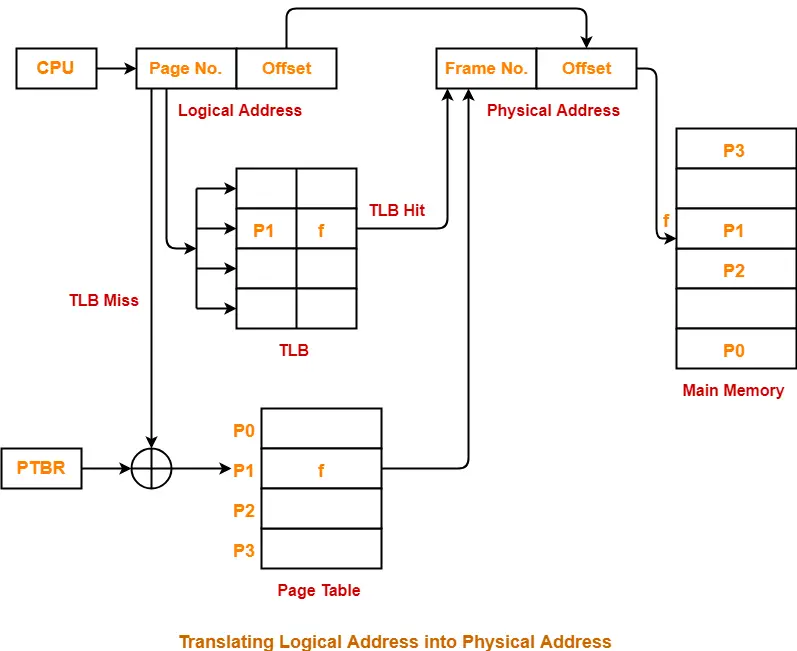## Flowchart-

The following flowchart illustrates the above steps of translating logical address into physical address-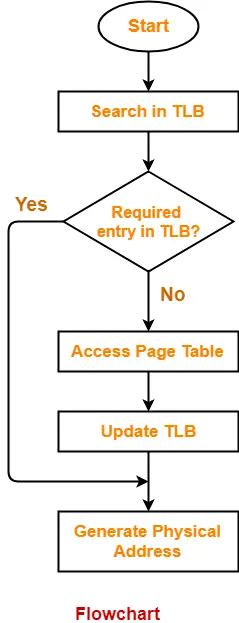## Important Points-

### Point-01:

• Unlike page table, there exists only one TLB in the system.
• So, whenever context switching occurs, the entire content of TLB is flushed and deleted.
• TLB is then again updated with the currently running process.

### Point-02:

When a new process gets scheduled-

• Initially, TLB is empty. So, TLB misses are frequent.
• With every access from the page table, TLB is updated.
• After some time, TLB hits increases and TLB misses reduces.

### Point-03:

• The time taken to update TLB after getting the frame number from the page table is negligible.
• Also, TLB is updated in parallel while fetching the word from the main memory.

The advantages of using TLB are-

• TLB reduces the effective access time.
• Only one memory access is required when TLB hit occurs.

A major disadvantage of using TLB is-

• After some time of running the process, when TLB hits increases and process starts to run smoothly, a context switching occurs.
• The entire content of the TLB is flushed.
• Then, TLB is again updated with the currently running process.

This happens again and again.

• TLB can hold the data of only one process at a time.
• When context switches occur frequently, the performance of TLB degrades due to low hit ratio.
• As it is a special hardware, it involves additional cost.

## Effective Access Time-

In a single level paging using TLB, the effective access time is given as-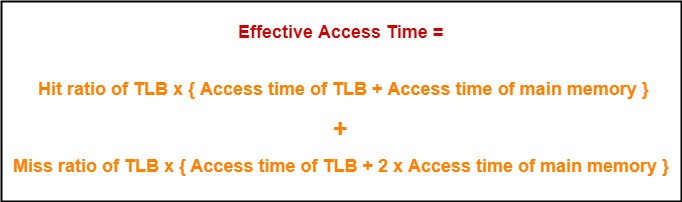This formula is valid only when there is single level paging and there are no page faults.

## Problem-01:

A paging scheme uses a Translation Lookaside buffer (TLB). A TLB access takes 10 ns and a main memory access takes 50 ns. What is the effective access time (in ns) if the TLB hit ratio is 90% and there is no page fault?

1. 54
2. 60
3. 65
4. 75

## Solution-

Given-

• TLB access time = 10 ns
• Main memory access time = 50 ns
• TLB Hit ratio = 90% = 0.9

### Calculating TLB Miss Ratio-

TLB Miss ratio

= 1 – TLB Hit ratio

= 1 – 0.9

= 0.1

### Calculating Effective Access Time-

Substituting values in the above formula, we get-

Effective Access Time

= 0.9 x { 10 ns + 50 ns } + 0.1 x { 10 ns + 2 x 50 ns }

= 0.9 x 60 ns + 0.1 x 110 ns

= 54 ns + 11 ns

= 65 ns

Thus, Option (C) is correct.

## Problem-02:

A paging scheme uses a Translation Lookaside buffer (TLB). The effective memory access takes 160 ns and a main memory access takes 100 ns. What is the TLB access time (in ns) if the TLB hit ratio is 60% and there is no page fault?

1. 54
2. 60
3. 20
4. 75

## Solution-

Given-

• Effective access time = 160 ns
• Main memory access time = 100 ns
• TLB Hit ratio = 60% = 0.6

### Calculating TLB Miss Ratio-

TLB Miss ratio

= 1 – TLB Hit ratio

= 1 – 0.6

= 0.4

### Calculating TLB Access Time-

Let TLB access time = T ns.

Substituting values in the above formula, we get-

160 ns = 0.6 x { T + 100 ns } + 0.4 x { T + 2 x 100 ns }

160 = 0.6 x T + 60 + 0.4 x T + 80

160 = T + 140

T = 160 – 140

T = 20

Thus, Option (C) is correct.

To gain better understanding about Translation Lookaside Buffer (TLB),

Watch this Video Lecture

Next Article- Multilevel Paging

Get more notes and other study material of Operating System.

Watch video lectures by visiting our YouTube channel LearnVidFun.